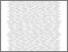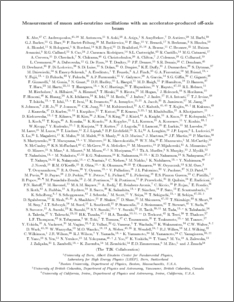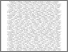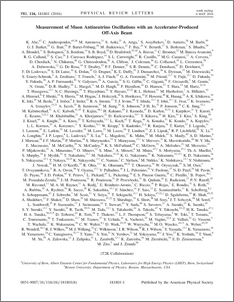# Measurement of muon antineutrino oscillations with an accelerator-produced off-axis beam

, T2K Collaboration (2016) Measurement of muon antineutrino oscillations with an accelerator-produced off-axis beam. Physical review letters, 116 (18). ISSN 1079-7114Preview
PDF (main)
main.pdf - Accepted VersionPreview
PDF (PhysRevLett.116.181801)
PhysRevLett.116.181801.pdf - Published Version

## Abstract

T2K reports its first measurements of the parameters governing the disappearance of $\overline{\nu}_\mu$ in an off-axis beam due to flavour change induced by neutrino oscillations. The quasi-monochromatic $\overline{\nu}_\mu$ beam, produced with a peak energy of 0.6 GeV at J-PARC, is observed at the far detector Super-Kamiokande, 295 km away, where the $\overline{\nu}_\mu$ survival probability is expected to be minimal. Using a dataset corresponding to $4.01 \times 10^{20}$ protons on target, $34$ fully contained $\mu$-like events were observed. The best-fit oscillation parameters are: $\sin^2 (\overline{\theta}_{23}) = 0.45$ and $|\Delta\overline{m}^2_{32}| = 2.51 \times 10^{-3}$ eV$^2$ with 68% confidence intervals of 0.38 - 0.64 and 2.26 - 2.80 ($\times 10^{-3}$ eV$^2$) respectively. These world-leading results are in agreement with existing anti-neutrino parameter measurements and also with the $\nu_\mu$ disappearance parameters measured by T2K.

Item Type:
Journal Article
Journal or Publication Title:
Physical review letters
8 pages, 4 figures
Uncontrolled Keywords:
/dk/atira/pure/subjectarea/asjc/3100
Subjects:
Departments:
ID Code:
78713
Deposited By:
Deposited On:
14 Mar 2016 09:02
Refereed?:
Yes
Published?:
Published